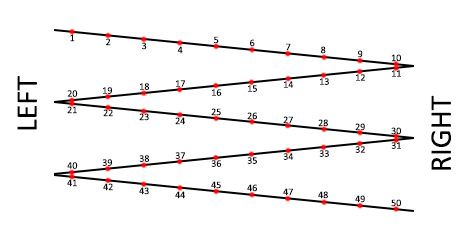# Math Question ... on string.

Some days I wish I took higher level math, but at the same time ... I probably wouldn't have the same amount of hair left on my noggin'. So, let's say you have a string of 100 LEDs. Now lay them down in a zig-zag pattern of 10 pixels each. Now comes the fun part, addressing the string. When laying the string down in a zig-zag pattern, let's say there's a left and a right, like this:NOTE: only showing 50 here, same thing for 100 or more.

Now, sending data to the string is easy, addressing the individual pixels, easy. But what I'm trying to figure out is, how do I calculate how data is send so that pixel numbers 1, 20, 21, 40, 41, etc., are considered to be the beginning of each segment (like a virtual pixel 0), and pixel numbers 10, 11, 30, 31, 50, 51, etc., are considered the end of each segment (virtual pixel 9).

Like, if I want a chase sequence, I don't want it to run from the first physical pixel to the last physical one. I want the sequence to run from the left to the right, on all the segments. So basically the information for the first segment needs to get reversed for the second segment.

Is there a mathematical way of doing this? Like, I can set two variables, one determining the physical length of the whole string, and one setting the length of each segment, then have the math take over?

I realize i can physically cut the string into ten pieces and address each one separately, but that's not the goal here. The goal is to have one string, one SPI port, and have the math take over.

KirAsh4:
Is there a mathematical way of doing this? Like, I can set two variables, one determining the physical length of the whole string, and one setting the length of each segment, then have the math take over?

It's called 'division'.

Divide the total number of LEDs by 10 (or whatever the segment length is).

And then what? How does the data being send out get re-ordered?

KirAsh4:
And then what? How does the data being send out get re-ordered?

Divide by ten and see if you get an odd or even number...

Yeah sorry. You're not making any sense. Breaking up the string isn't the problem. Reordering the data is.

Divide by ten. If you get an even number (or zero) the segment goes right, if you get an odd number it goes left.